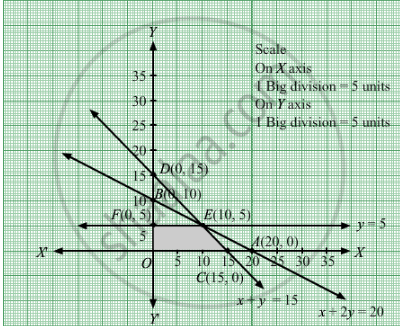# Maximize-z-3x-5y-subject-x-2-y-20-x-y-15-y-5-x-y-0 - Mathematics

Maximize Z = 3x + 5y
Subject to

$x + 2y \leq 20$
$x + y \leq 15$
$y \leq 5$
$x, y \geq 0$

#### Solution

We need to maximize Z = 3x + 5y

First, we will convert the given inequations into equations, we obtain the following equations:
x + 2y = 20, x + y = 15, y = 5 , x = 0 and y = 0.

The line x + 2y = 20 meets the coordinate axis at A(20, 0) and B(0,10). Join these points to obtain the line x + 2y = 20.
Clearly, (0, 0) satisfies the inequation x + 2y ≤ 20. So, the region in xy-plane that contains the origin represents the solution set of the given equation.

The line x + y = 15 meets the coordinate axis at C(15, 0) and D(0,15). Join these points to obtain the line x + y = 15.
Clearly, (0, 0) satisfies the inequation x + ≤ 15. So, the region in xy-plane that contains the origin represents the solution set of the given equation.

y = 5 is the line passing through (0, 5) and parallel to the X axis.The region below the line y = 5 will satisfy the given inequation.

Region represented by x ≥ 0 and y ≥ 0:
Since, every point in the first quadrant satisfies these inequations. So, the first quadrant is the region represented by the inequations.
These lines are drawn using a suitable scale.The corner points of the feasible region are O(0, 0), $C\left( 15, 0 \right)$ , $E\left( 10, 5 \right)$ and $F\left( 0, 5 \right)$ The values of Z at these corner points are as follows.

 Corner point Z = 3x + 5y O(0, 0) 3 × 0 + 5 × 0 = 0 $C\left( 15, 0 \right)$ 3 × 15 + 5 × 0 = 45 $E\left( 10, 5 \right)$ 3 × 10 + 5 × 5 = 55 $F\left( 0, 5 \right)$ 3 × 0 + 5 × 5 = 25

We see that the maximum value of the objective function Z is 55 which is at $E\left( 10, 5 \right)$

Thus, the optimal value of Z is 55.

Concept: Graphical Method of Solving Linear Programming Problems
Is there an error in this question or solution?

#### APPEARS IN

RD Sharma Class 12 Maths
Chapter 30 Linear programming
Exercise 30.2 | Q 15 | Page 32